# Introduction To Heat Flow

Heat energy usually flows from a higher energy body to a lower energy body. This quiz contains various questions regarding the concept of heat flow. Different questions are asked in the quiz to let students learn the concept of heat flow easily and effectively.

Start Quiz

Which figure shows the correct direction of heat flow?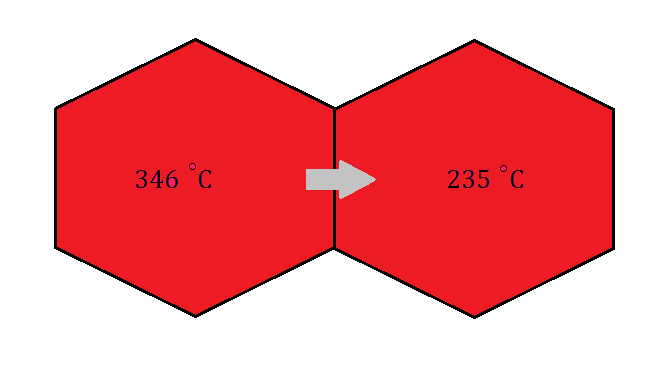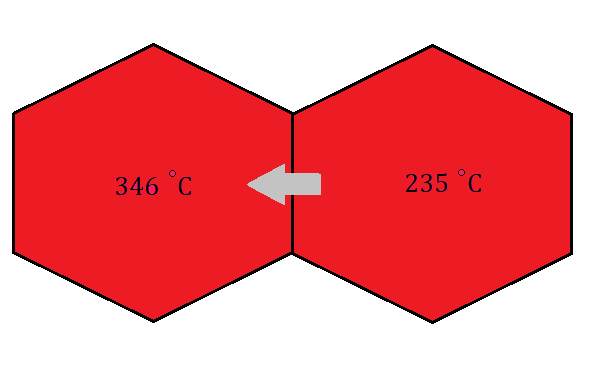Which figure shows the correct direction of heat flow?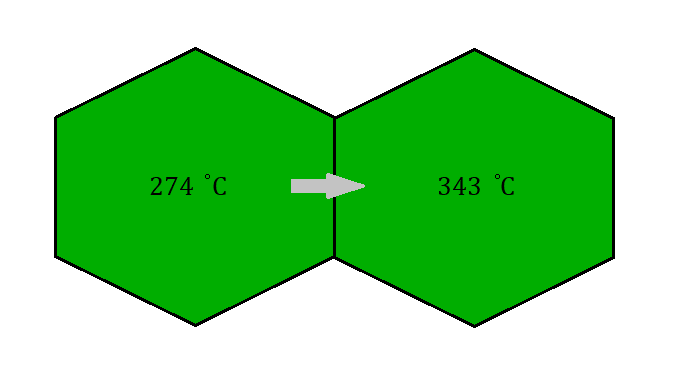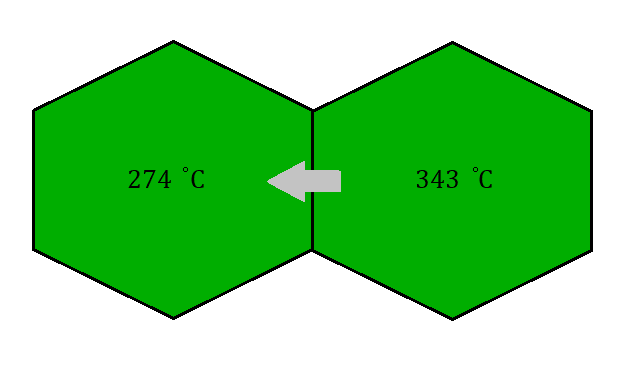Which figure shows the correct direction of heat flow?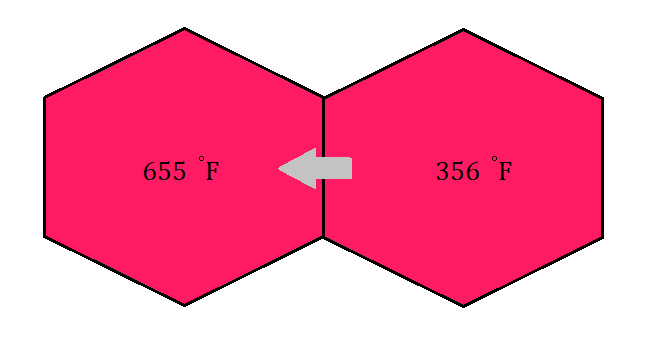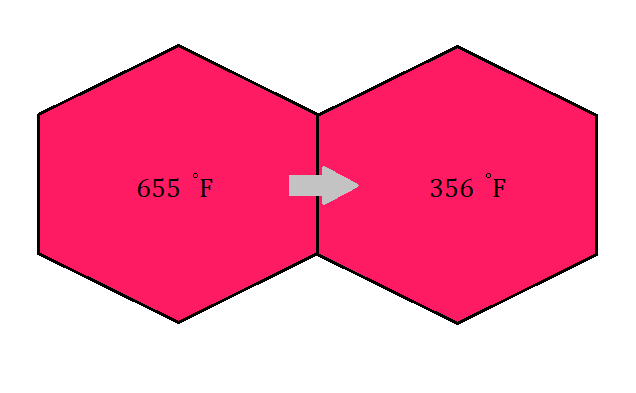Is the direction of heat flow shown in the figure correct or incorrect?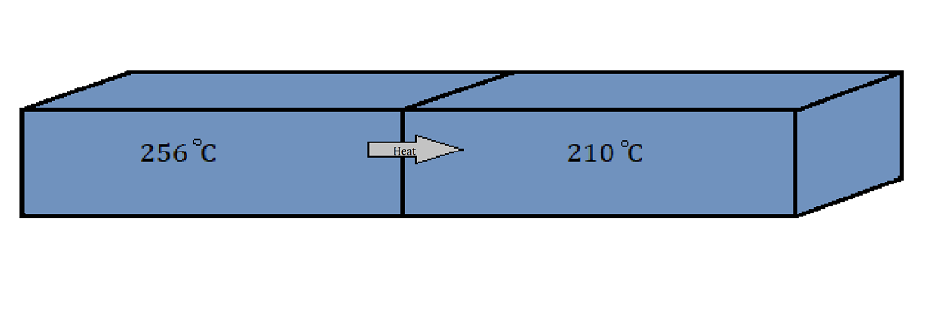Correct

Incorrect

Is the direction of heat flow shown in the figure correct or incorrect?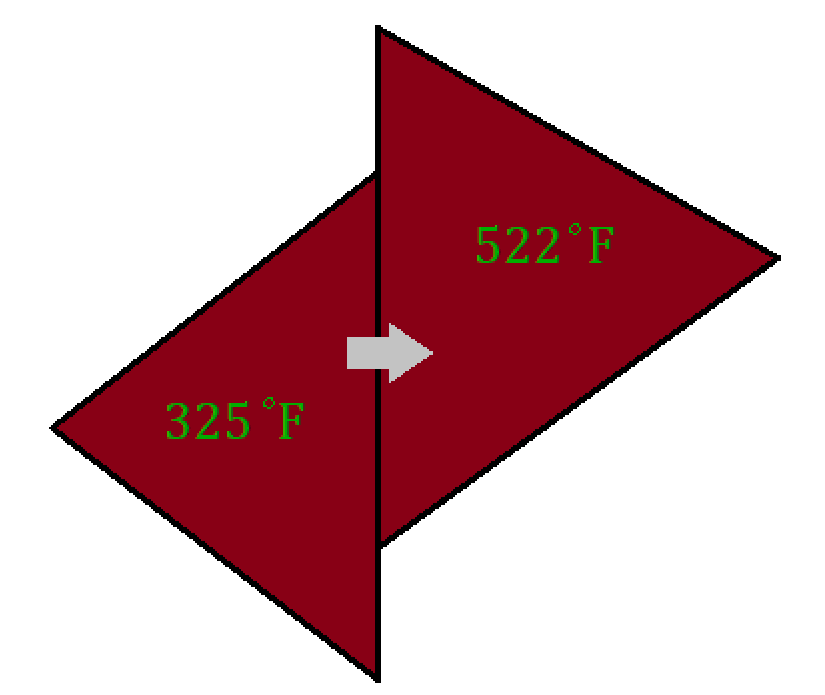Correct

Incorrect

Is the direction of heat flow shown in the figure correct or incorrect?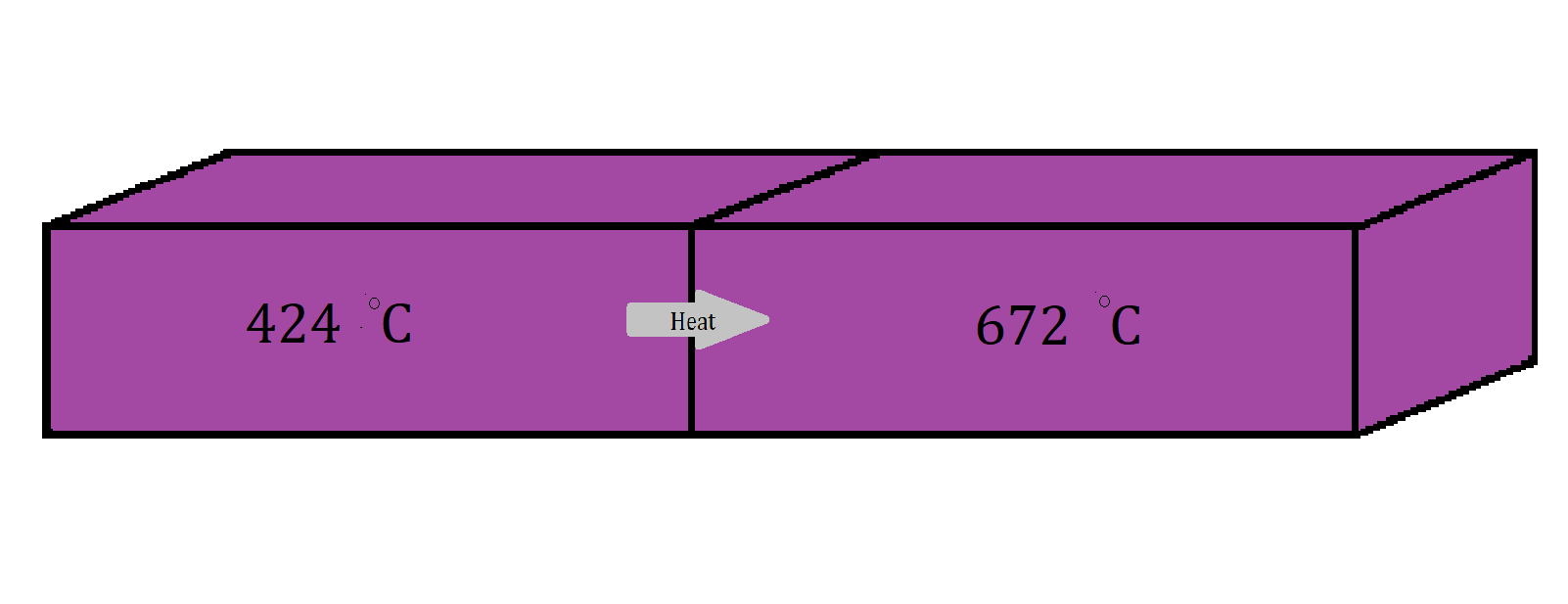Correct

Incorrect

Quiz/Test Summary
Title: Introduction To Heat Flow
Questions: 6
Contributed by: